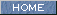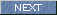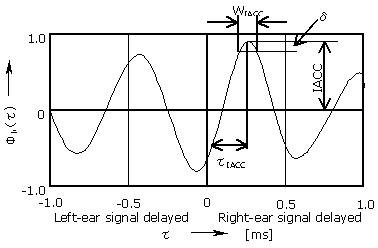| Japanese | English |

### Analysis of the sound source direction from the cross-correlation  (Automobile Noise Analysis Test 6)

In car5.htm, arrival directions of sound from the engine and two mufflers were analyzed with the short analysis time of 0.2 second. To advance the analysis further, the same data is analyzed by shortening analysis time.

Before showing the results, some background knowledge about the analysis method is introduced.

Directional information is analyzed by the parameter tIACC, which is obtained from the two channel cross-correlation function. This parameter is defined in the figure below.The tIACC is represented in delay time (ms) of the cross-correlation function. When the peak of the cross-correlation is at the position of the zero of the X-axis, a sound source is in the front of microphones. If the sound source is in right, sound will reach the right microphone early. In this case, tIACC becomes positive.

The right-hand side (positive mark) of the X-axis is the delay time of the sound signal of a right channel. Left-hand side (negative mark) is the delay time of a left channel. The value of the Y-axis expresses the degree of the cross-correlation of the sound signals of a right channel and a left channel.

Divide the distance difference between two microphones and a sound source by the sound speed to obtain the time difference between two microphones (tIACC). As shown in a figure below, let us consider a right-angled triangle. When a interval between two microphones is 2.5 cm and the angle between the sound source and microphones are 30 degrees or 60 degrees, remaining lengths are 2cm and 1.5cm. Suppose that the sound speed is 340m/s. Then the time difference for 30 degrees becomes 0.015(m)/340(m/s)*1000=0.044(msec).Figure 1 shows the case that the sound source is in the front of microphones. The delay time is zero.

Figure 2 shows that the sound source is at 30 degrees in the right direction. Time delay is 0.044msec in this case.

Figure 3 shows that the sound source is at 60 degrees in the right direction. Time delay is 0.058msec in this case.

When the delay time of Left CH is 0.073msec, sound source is in the direction of 90 degrees.

The arrival direction of sound can be calculated from the delay time of this tIACC using the stereo microphone whose microphone distance is less than 2cm. In the case that the distance is 2 cm, the range of the tIACC is -0.06 ms < tIACC < +0.06 ms.

Generally, tIACC is defined in the range between ±1.0 ms considering the distance between right ear and left ear of human head. To calculate the angle of the sound source from the inter-aural time difference for the human head, the effect of the diffraction, scattering, interference of the ear and the head should also be considered.

April 2003 by Masatsugu Sakurai